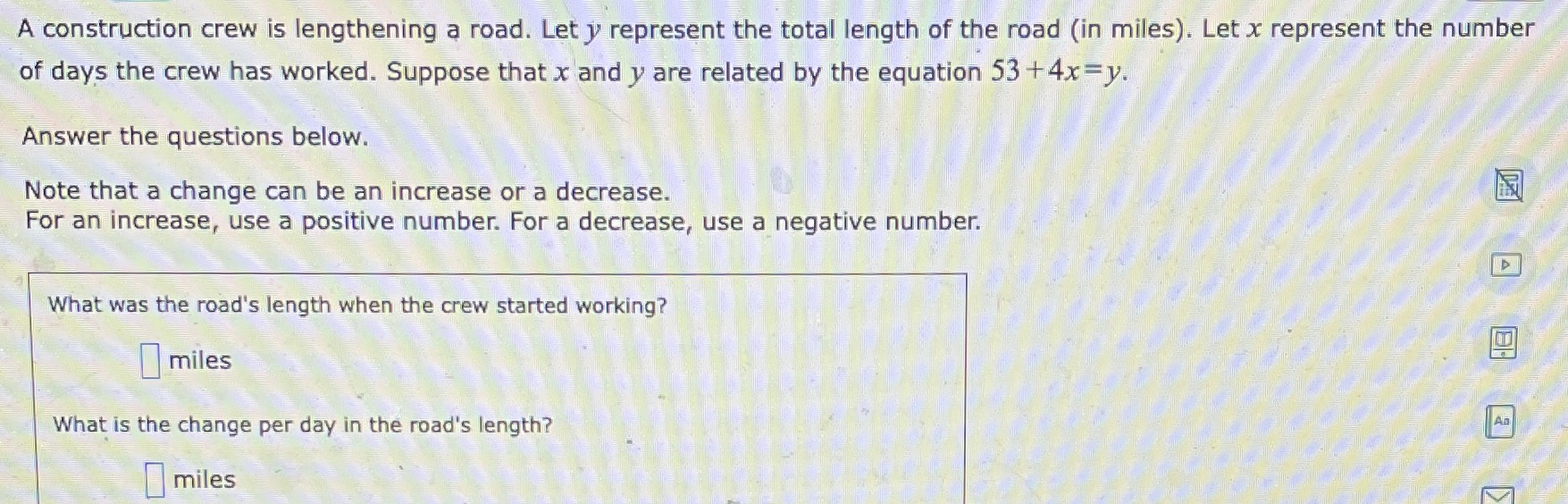### ¿Todavía tienes preguntas de matemáticas?

Pregunte a nuestros tutores expertos
Algebra
PreguntaA construction crew is lengthening a road. Let $$y$$ represent the total length of the road (in miles). Let $$x$$ represent the number of days the crew has worked. Suppose that $$x$$ and $$y$$ are related by the equation $$53 + 4 x = y$$ . Answer the questions below. Note that a change can be an increase or a decrease. For an increase, use a positive number. For a decrease, use a negative number. What was the road's length when the crew started working? miles